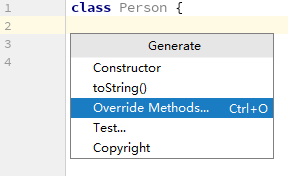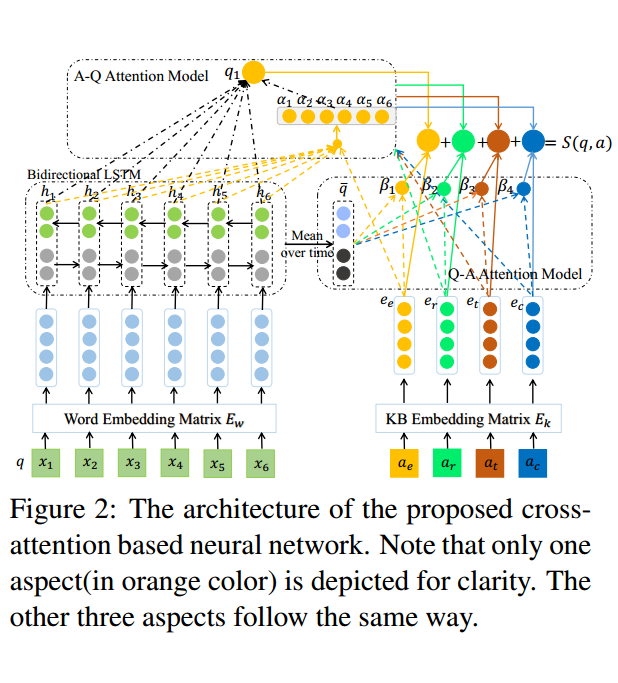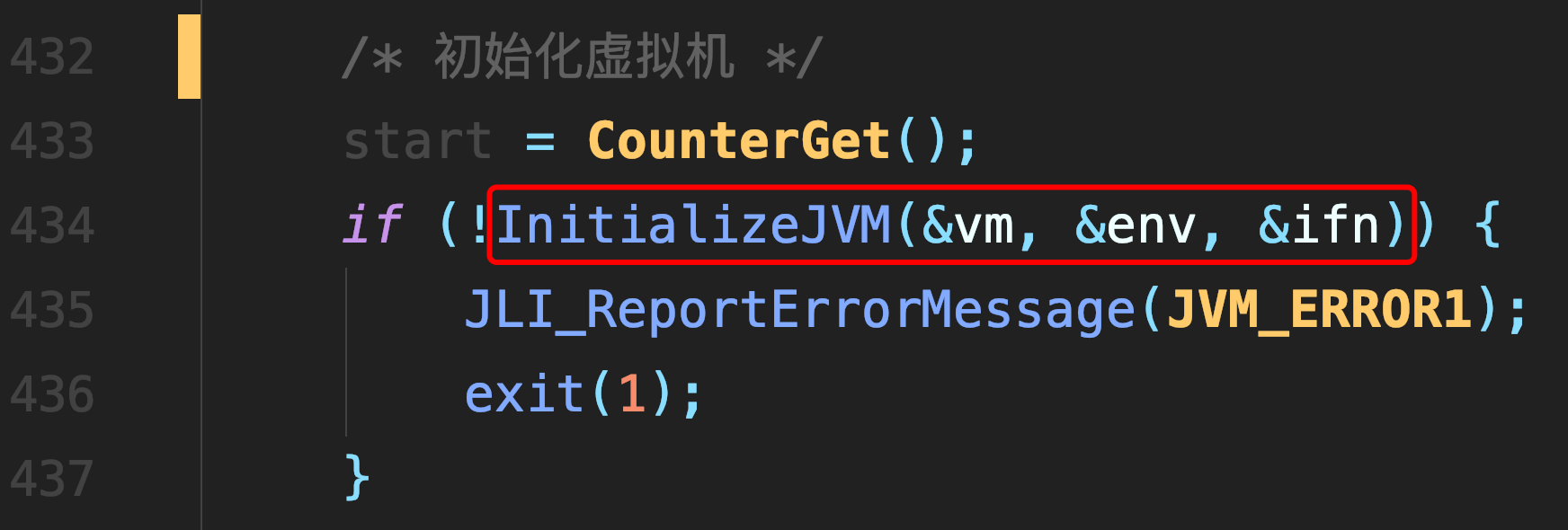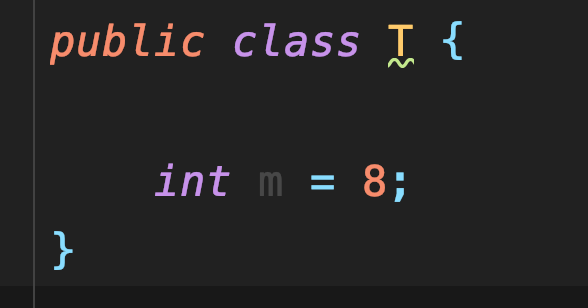# 3 迭代器 生成器

### 迭代器

```# 迭代：是一个重复的过程，每一次重复，都是基于上一次的结果的而来

# while True:      # 单纯的重复
print('你瞅啥')

l = ['a','b','c','d']

count =0
while count < len(l):
print(l[count])
count+=1```

不依赖于索引 ... 更省内存空间...

一次性，只能往后走，不能回头，不如索引灵活。

不能预知长度，无法预知什么时候结束 。。。。

现接触的有     str  '  '... list   [ ]  ... tuple   (  ) ..... set  {  }  .... dict  { key:vaule }  ... f = open( 'db.txt ')

```#1、为何要有迭代器？

#2、什么是可迭代对象？

'hello'.__iter__
(1,2,3).__iter__
[1,2,3].__iter__
{'a':1}.__iter__
{'a','b'}.__iter__
open('a.txt').__iter__

#3、什么是迭代器对象？

open('a.txt').__iter__()
open('a.txt').__next__()

#4、注意：

`迭代器对象的优点1：提供了一种统一的（不依赖于索引的）迭代方式2：迭代器本身，比起其他数据类型更省内存，因为同一时间只next一个值，在内存中。`
`迭代器对象的缺点1:一次性，只能往后走，不能回退，不如索引取值灵活2：无法预知什么时候取值结束，即无法预知长度````dic={'a':1,'b':2,'c':3}
iter_dic=dic.__iter__() #得到迭代器对象，迭代器对象即有__iter__又有__next__，但是：迭代器.__iter__()得到的仍然是迭代器本身
iter_dic.__iter__() is iter_dic #True

print(iter_dic.__next__()) #等同于next(iter_dic)
print(iter_dic.__next__()) #等同于next(iter_dic)
print(iter_dic.__next__()) #等同于next(iter_dic)
# print(iter_dic.__next__()) #抛出异常StopIteration，或者说结束标志

#有了迭代器，我们就可以不依赖索引迭代取值了
iter_dic=dic.__iter__()
while 1:
try:
k=next(iter_dic)
print(dic[k])
except StopIteration:
break

#这么写太丑陋了，需要我们自己捕捉异常，控制next，python这么牛逼，能不能帮我解决呢？能，请看for循环```### from collections import Iterable,Iterator  判断是否可迭代对象 or 迭代器对象 ..... 了解部分

iterable 　　可迭代对象 返回 True  .....

iterator　　  迭代器对象 返回 True  .....for循环

```#基于for循环，我们可以完全不再依赖索引去取值了
dic={'a':1,'b':2,'c':3}
for k in dic:
print(dic[k])

#for循环的工作原理
#1：执行in后对象的dic.__iter__()方法，得到一个迭代器对象iter_dic
#2: 执行next(iter_dic),将得到的值赋值给k,然后执行循环体代码
#3: 重复过程2，直到捕捉到异常StopIteration,结束循环```
```l=['a','b','c','d']
for item in l: #iter_l=l.__iter__()
print(item)

for item in {1,2,3,4}:
print(item)

with open('a.txt') as f:
for line in f: #i=f.__iter__()
print(line)
print(f is f.__iter__())```

### 生成器

只要函数内部包含yield 关键字 ，那么函数名()的到的结果就是生成器，并且不会执行函数内部代码```#只要函数内部包含有yield关键字，那么函数名()的到的结果就是生成器，并且不会执行函数内部代码

def func():
print('====>first')
yield 1
print('====>second')
yield 2
print('====>third')
yield 3
print('====>end')

g=func()
print(g) #<generator object func at 0x0000000002184360>

# generator ```

```g.__iter__
g.__next__
#2、所以生成器就是迭代器，因此可以这么取值
res=next(g)
print(res)```

```#!/usr/bin/env python3
# _*_ coding:utf-8 _*_
# @File    : 生成器
# @Version : 1.0

from collections import Iterator

'''
生成器：
在函数内部包含yield关键字，那么该函数执行结果就是生成器（但是生成器本身没有值，只有next时才有值）

生成器就是迭代器

yield 功能：

将函数的结果做成生成器（以一种优雅的方式封装好 __iter__ 和 __next__）

函数暂停与再继续运行由  yield 控制

'''

def foo():
print('1')
yield 'first'
print('2')
yield 'second'
print('3')
yield 'third'
print('4')
yield 'fourth'
print('5')
yield 'fifth'
print('6')
yield 'sixth'
print('7')
yield 'seventh'

g = foo()                           #   它的名字就做 generator

print(g)                            #   < Generator object at ..... >

print(isinstance(g,Iterator))       # True     一定有  g.__iter__   and g.__next__

print(next(g))
print(next(g))
print(next(g))

for item in g:
print(item)

print(100*'{0}'.format('#'))
# -----------------------------------------------------------------------------------------------

def bar(n):                         # 产生一个无限迭代的生成器
while True:
print('begin....')
yield n
n+=1

g1 = bar(0)
print(isinstance(g1,Iterator))
print(next(g1))
print(next(g1))
print(next(g1))

'''
for k in g1:
if k == 100:break
print(k)
'''

'''
for 遍历迭代器的好处就是不用手动处理异常。迭代器 next 没有值时会抛出 StopIteration 异常,
----    for 循环主动获取异常后终止遍历，迭代器取值完毕。

'''

print(100*'{0}'.format('#'))
# -----------------------------------------------------------------------------------------------

def my_range(start,stop):
while True:
if start == stop:
raise StopIteration
yield start
start +=1

g3 = my_range(9,90)

print(next(g3))

for k in g3:
print(k)

'''
生成器模仿range功能， while 循环内 加上 if 判断,条件成立时主动抛出异常，迭代器终止,否则会是一个无限迭代。
raise --主动抛出异常    -- 后面跟 异常类型

'''```

##### 热门推荐
•浏览(649)
•浏览(556)
•浏览(540)
•浏览(468)
•浏览(423)
•浏览(402)
•浏览(379)
•浏览(365)
•浏览(357)
•浏览(315)